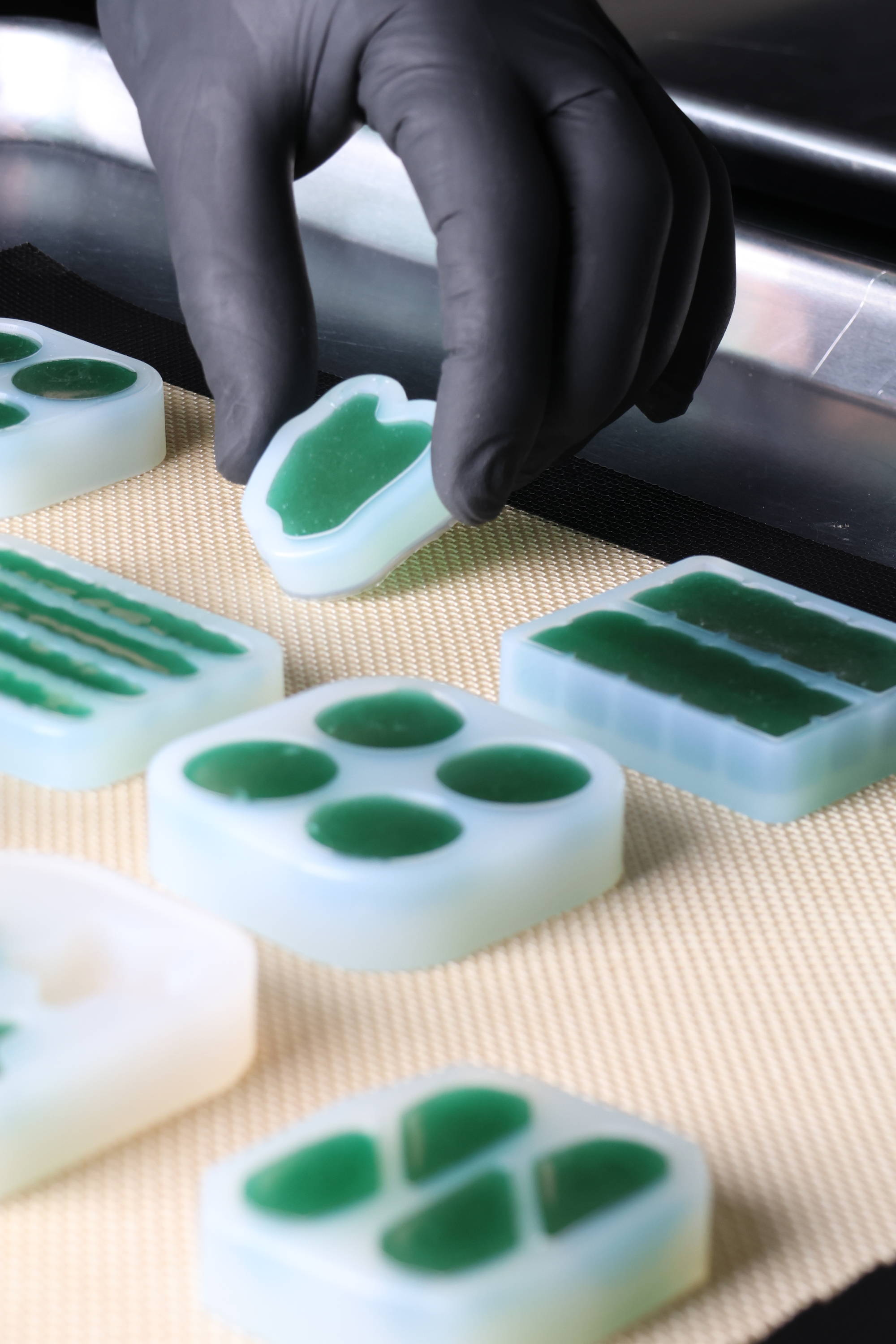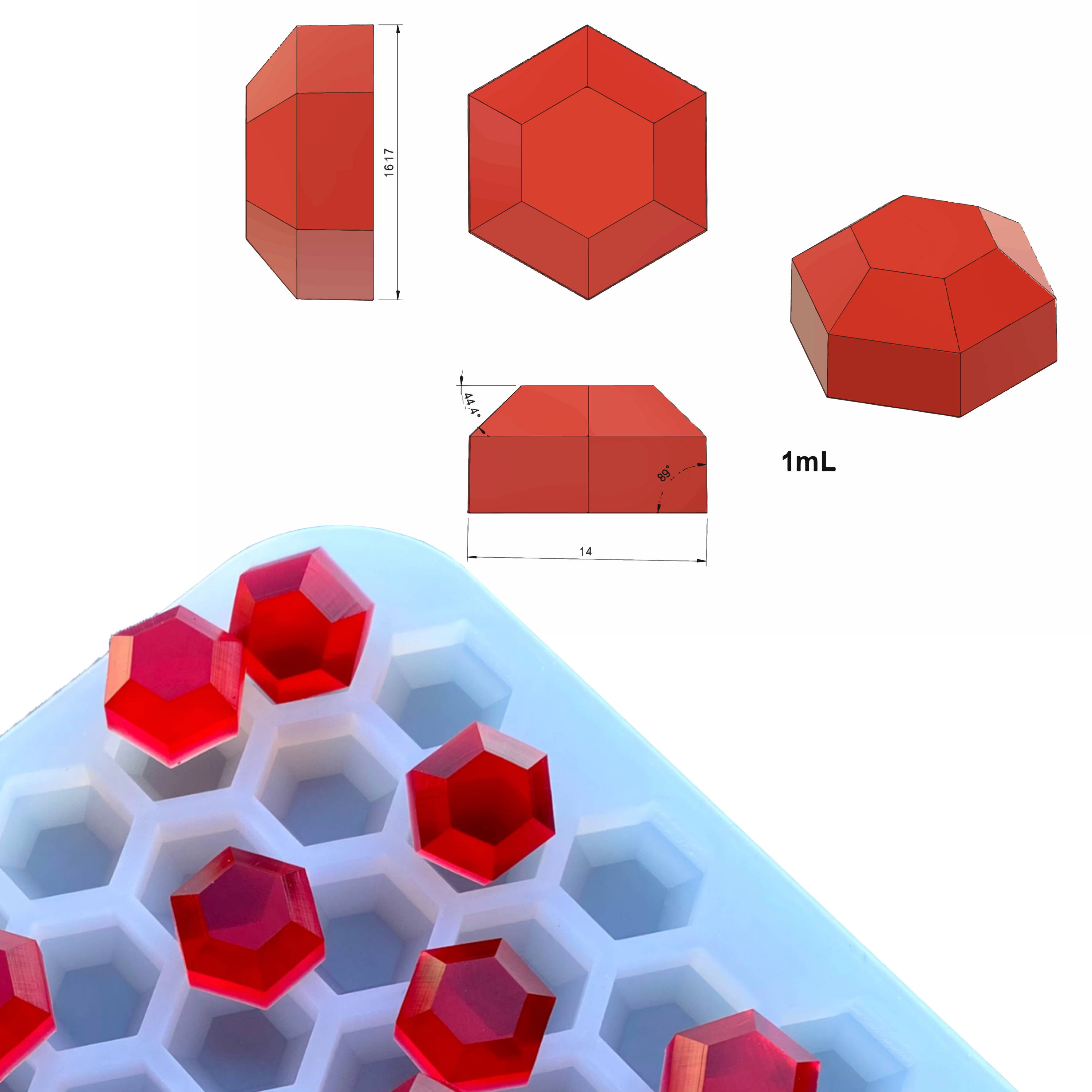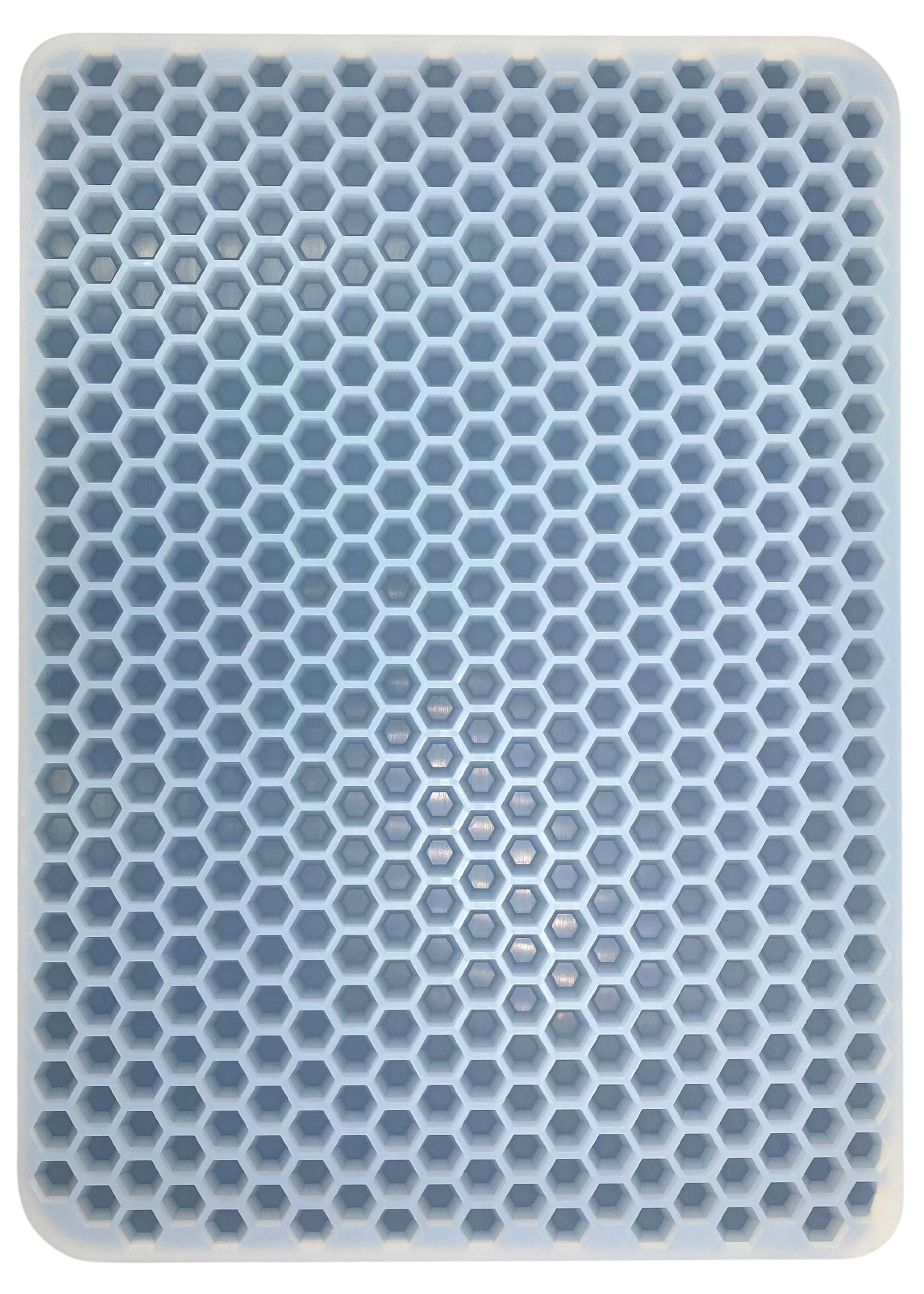# cALCULATE WEIGHT

Need to figure out the weight of your gummy by the volume?

A typical gummy is about 80-82 Brix (% total solids), as measured on a refractometer at room temperature.

The weight is about 1.4 the weight of water, for the same volume.

So multiply the volume of your mold cavity by 1.4 to get the weight of a gummy from that mold.

Example: 3 ml x 1.4 = 4.2 gram gummy## Example for Cavity Calculation weightMeasure with water your cavity volume, we recommend you to use a syringe. If you buy from Vector Molds make sure to note the cavity volume.
In this example the cavity volume is 1 mL

Make fun calculations

With the 1.4 density gummy factor multiply the cavity volume.
in this case 1 mL x 1.4 g/mL = 1.4 g

Weight of a single cavity

Perfect! Now we know that the weigh of a single cavity is 1.4g.

### Example For Full Mold Calculation Weight

Weight of the full candy mold

Now multiply your 1.4 g per the number of cavities. In this case 1.4 g x 478 cavities= 669.2g